Maths-
General
Easy

Question

# The ratio of the bases of two triangles is a : b. If the ratio of their corresponding altitudes is c : d, find the ratio of their areas (in the same order).Hint:

## The correct answer is: ac : bd

### It is given that ratio of bases of two triangle is a : b⇒ Bases of the triangles = ax , bxSimilarly, it is given that ratio of altitudes is c : d⇒ Altitudes of the triangle = cy , dyArea of first triangle =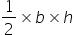=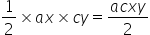Area of second triangle =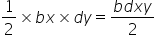Ratio of the areas of two triangle=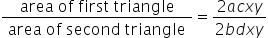=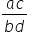Hence, ratio of area of two triangles is ac : bd#### With Turito Foundation.#### Get an Expert Advice From Turito.